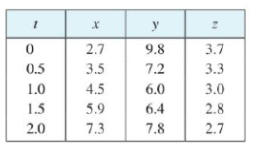Chapter 13.4, Problem 1E

Chapter
Section
Textbook Problem

# The table gives coordinates of a particle moving through space along a smooth curve.(a) Find the average velocities over the time intervals [0, 1), [0.5, 1], [1, 2], and [1, 1.5].(b) Estimate the velocity and speed of the particle at t = 1.(a)

To determine

To find: The average velocities over the time interval [0,1].

Explanation

Given:

The coordinates of particle moving through space along a smooth curve is given in Table 1.

Table 1

 t x y z 0 2.7 9.8 3.7 0.5 3.5 7.2 3.3 1.0 4.5 6.0 3.0 1.5 5.9 6.4 2.8 2.0 7.3 7.8 2.7

Formula used:

Write the general expression to find the average velocity over the time [t1,t2].

vave=r(t2)r(t1)t2t1 (1)

The position vector of the particle is,

r(t)=x(t)i+y(t)j+z(t)k (2)

At t=0.

Substitute 0 for t in equation (2),

r(0)=x(0)i+y(0)j+z(0)k

Substitute 2.7 for x(0), 9.8 for y(0) and 3.7 for z(0) from the given table,

r(0)=2.7i+9.8j+3.7k

At t=1.

Substitute 1 for t in equation (2),

r(1)=x(1)i+y(1)j+z(1)k

Substitute 4.5 for x(1), 6

(b)

To determine

To estimate: The velocity of the particle at t=1.

### Still sussing out bartleby?

Check out a sample textbook solution.

See a sample solution

#### The Solution to Your Study Problems

Bartleby provides explanations to thousands of textbook problems written by our experts, many with advanced degrees!

Get Started

#### Find more solutions based on key concepts